# Optical Fields and Maxwell's Equations

Photonics is an engineering discipline concerning the control of light, or photons, for useful applications, much as electronics has to do with electrons. Light is electromagnetic radiation of frequencies in the range from 1 THz to 10 PHz, corresponding to wavelengths between ~300 μm and ~30 nm in free space. This optical spectral range is generally divided into infrared, visible, and ultraviolet regions, as indicated in the table below.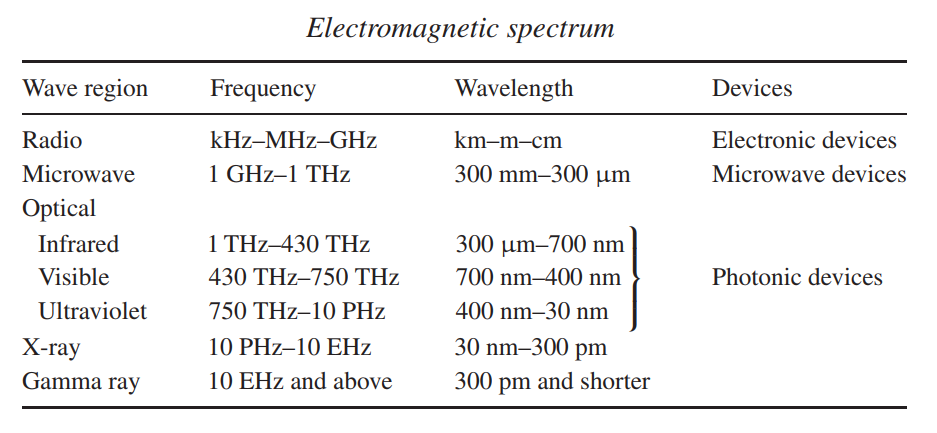The spectral range of concern in photonics is usually in a wavelength range between 10 μm and 100 nm. The primary interest in the applications of photonic devices is in an even narrower range of visible and near-infrared wavelengths. As we shall see later, this spectral range of application is largely determined by the properties of materials used for photonic devices.

The wave nature of light is very important in the function of photonic devices. In particular, the propagation of light in a photonic device is completely characterized by its wave nature. However, in the spectral range of interest for practical photonic devices, the quantum energies of photons are in a range where the quantum nature of light is also important. For example, photons of visible light have energies between 1.7 and 3.1 eV, which are in the range of the bandgaps of most semiconductors. Photon energy is an important factor that determines the behavior of an optical wave in a semiconductor photonic device. The uniqueness of photonic devices is that both wave and quantum characteristics of light have to be considered for the function and applications of these devices. Generally speaking, the photon nature of light is important in the operation of photonic devices for generation, amplification, frequency conversion, or detection of light, while the wave nature is important in the operation of all photonic devices but is particularly so for devices used in transmission, modulation, or switching of light. In this tutorial, we review some relevant wave and quantum properties of light as a general background for later tutorials.

Optical Fields and Maxwell's Equations

When dealing with photonic devices, we consider in most situations optical fields in media of various electromagnetic properties. The electromagnetic field in a medium is generally characterized by the following four field quantities:

electric field                          E(r, t)           V m-1

electric displacement            D(r,t)            C m-2

magnetic field                       H(r, t)            A m-1

magnetic induction                B(r, r)           T or Wb m-2

Note that E and B are fundamental microscopic fields, while D and H are macroscopic fields that include the response of the medium. The units given above and below for the field quantities are SI units consistent with the SI system used in this series of tutorials for Maxwell's equations.

Experimentally measured magnetic field quantities are sometimes given in Gaussian units, which are gauss for the B field and oersted (Oe) for the H field. The conversion relations between SI and Gaussian units are 1 T = 1 Wb m-2 = 10-4 gauss for B and 1 A m-1 = 4π x 10-3 Oe for H.

The response of a medium to an electromagnetic field generates the polarization and the magnetization:

polarization (electric polarization)            P(r, t)        C m-2

magnetization (magnetic polarization)     M(r, t)        A m-1

They are connected to the field quantities through the following relations:

D(r, t) = ε0E(r, t) + P(r, t)                                                                                     (1)

and

B(r, t) = μ0H(r, t) + μ0M(r, t)                                                                                (2)

where

ε0 = 1/(36π) x 10-9   F m-1 or A s V-1 m-1                                                            (3)

is the electric permittivity of free space and

μ0 = 4π x 10-7   H m-1 or V s A-1 m-1                                                                   (4)

is the magnetic permeability of free space. In addition, independent charge or current sources may exist:

charge density         ρ(r, t)     C m-3,

current density         J(r, t)      A m-2.

In a medium, the behavior of a time-varying electromagnetic field is governed by the following space- and time-dependent macroscopic Maxwell's equations: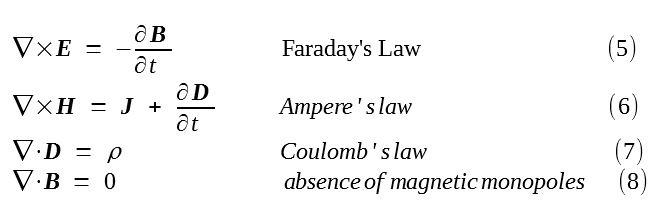The current and charge densities are constrained by the following continuity equation:In a medium free of sources, J = 0 and ρ = 0. Then, Maxwell's equations are simplyThese are equations normally used for optical fields because optical fields are usually not generated directly by free currents or free charges.

Transformation Properties

Maxwell's equations and the continuity equation are the basic physical laws that govern the behavior of electromagnetic fields. They are invariant under the transformation of space inversion, in which the spatial vector r is changed to r' = -r, or (x, y, z) → (-x, -y, -z), and the transformation of time reversal, in which the time variable t is changed to t' = -t, or t → -t. This means that the form of these equations is not changed when we perform the space-inversion transformation or the time-reversal transformation, or both together.

Different quantities in Maxwell's equations have different transformation properties. An understanding of these properties is important and leads to a fundamental appreciation of the difference between the characteristics of the electric and magnetic fields, which is the origin of the difference between the electric and magnetic symmetry properties of materials. It also helps in understanding many basic characteristics of the electro-optic, magneto-optic, and nonlinear optical properties of materials to be addressed in later tutorials.

The electric field vectors, E and D, have the same transformation properties as those of P, while the transformation properties of the magnetic field vectors, H and B, are the same as those of M

The origin of the electric properties of a material is the charge-density distribution, ρ(r, t), at the atomic level in the material, whereas that of the magnetic properties stems from the current-density distribution, J(r, t).

The transformation properties of the scalar quantity ρ are such that the sign of ρ remains unchanged under the transformation of either space inversion or time reversal.

In contrast, J is a polar vector because it is charge density times velocity, ρv, where velocity, v, is a polar vector. Thus, the vector J changes sign under the transformation of either space inversion or time reversal. It changes sign under space inversion because a polar vector changes sign under space inversion, and it changes sign under time reversal because v is the first time derivative of r.

The electric polarization P is a polar vector because it is the volume average of the electric dipole moment density defined by ρ(r, t)r, and the product of a scalar quantity ρ and a polar vector r is a polar vector.

In contrast, magnetization M is an axial vector because it is the volume average of the magnetic dipole moment density defined by r × J(r, t), and the cross product of two polar vectors, r and J, is an axial vector.

Therefore, we find the following transformation properties.

1. Electric fields.  The electric field vectors, P, E, and D, change sign under space inversion but not under time reversal.

2. Magnetic fields.  The magnetic field vectors, M, H, and B change sign under time reversal but not under space inversion.

With these transformation properties understood, the invariance of Maxwell's equations and the continuity equation under the transformation of space inversion or time-reversal or both can be easily verified.

Response of Medium

Polarization and magnetization in a medium are generated, respectively, by the response of the medium to the electric and magnetic fields. Therefore, P(r, t) depends on E(r, t), while M(r, t) depends on B(r, t). At optical frequencies, the magnetization vanishes, M = 0. Consequently, for optical fields, the following relation is always true:

B(r, t) = μ0H(r, t)                                         (14)

This is not true at low frequencies, however. It is possible to change the properties of a medium through a magnetization induced by a DC or low-frequency magnetic field, leading to the functioning of magneto-optic devices. It should be noted that even for magneto-optic devices, magnetization is induced by a DC or low-frequency magnetic field that is separate from the optical fields. No magnetization is induced by the magnetic components of the optical fields.

Except for magneto-optic devices, most photonic devices are made of dielectric materials that have zero magnetization at all frequencies. The optical properties of such materials are completely determined by the relation between P(r, t) and E(r, t). This relation is generally characterized by an electric susceptibility tensorχ, through the following definition for electric polarization: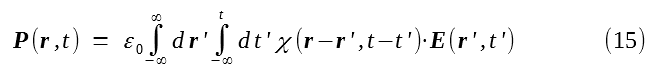From equation (1), then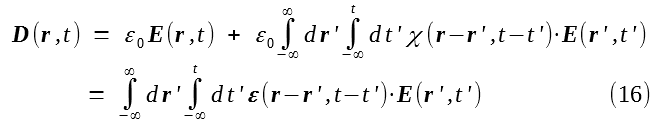where ε is the electric permittivity tensor of the medium.

Because χ and, equivalently, ε represent the response of a medium to the optical field and thus completely characterize the macroscopic electromagnetic properties of the medium, (15) and (16) can be regarded as the definitions of P(r, t) and D(r, t), respectively. A few remarks can be made:

1. Both χ and ε are generally tensors because the vectors P and D are, in general, not parallel to vector E due to material anisotropy. In the case of an isotropic medium, both χ and ε can be reduced to scalars χ and ε, respectively.

2. The relations in (15) and (16) are in the form of convolution integrals. The convolution in time accounts for the fact that the response of a medium to excitation of an electric field is generally not instantaneous or local in time and will not vanish for some time after the excitation is over. Because time is unidirectional, causality exists in physical processes. An earlier excitation can have an effect on the property of a medium at a later time, but not a later excitation on the property of the medium at an earlier time. Therefore, the upper limit in the time integral is t, not infinity. In contrast, the convolution in space accounts for the spatial nonlocality of the material response. Excitation of a medium at a location r' can result in a change in the property of the medium at another location r. For example, the property of a semiconductor at one location can be changed by electric or optical excitation at another location through carrier diffusion. Because space is not unidirectional, there is no spatial causality, in general, and spatial convolution is integrated over the entire space. Figure 1 below shows the temporal and spatial nonlocality of responses to electromagnetic excitations. The temporal nonlocality of the optical response of a medium results in frequency dispersion of its optical property, while the spatial nonlocality results in momentum dispersion.

3. In addition to the dependence on space and time through the convolution relation with the optical field, χ and ε can also be functions of space or time independent of the optical field because of spatial or temporal inhomogeneities in the medium. Spatial inhomogeneity exists in all optical structures, such as optical waveguides, where the index of refraction is a function of space. Temporal inhomogeneity exists when the optical property of a medium varies with time, for example, because of modulation by a low-frequency electric field or by an acoustic wave.

4. In a linear medium, χ and ε do not depend on the optical field E. In a nonlinear optical material, χ and ε are themselves also functions of E.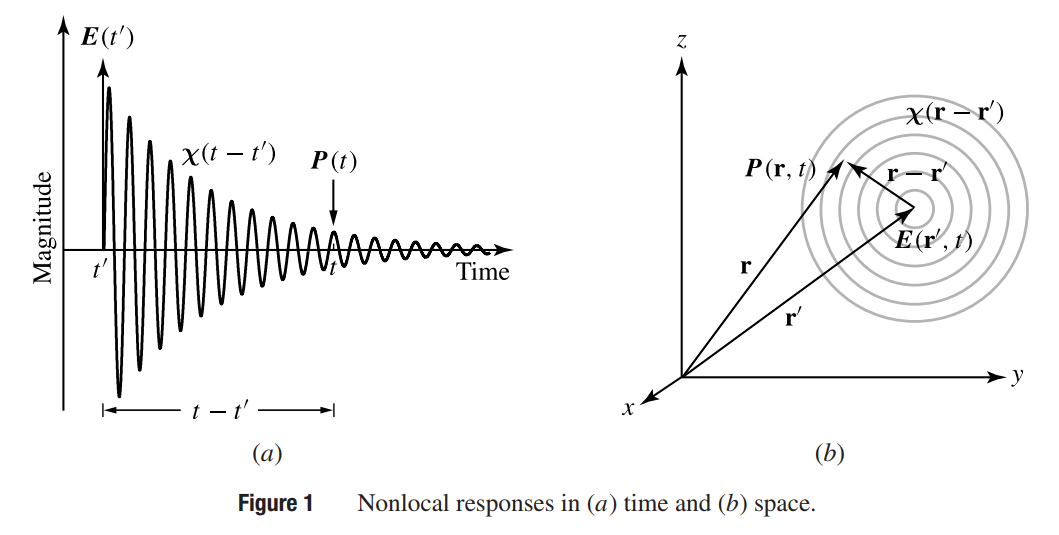Boundary Conditions

At the interface of two media of different optical properties, as shown in figure 2 below, the optical field components must satisfy certain boundary conditions.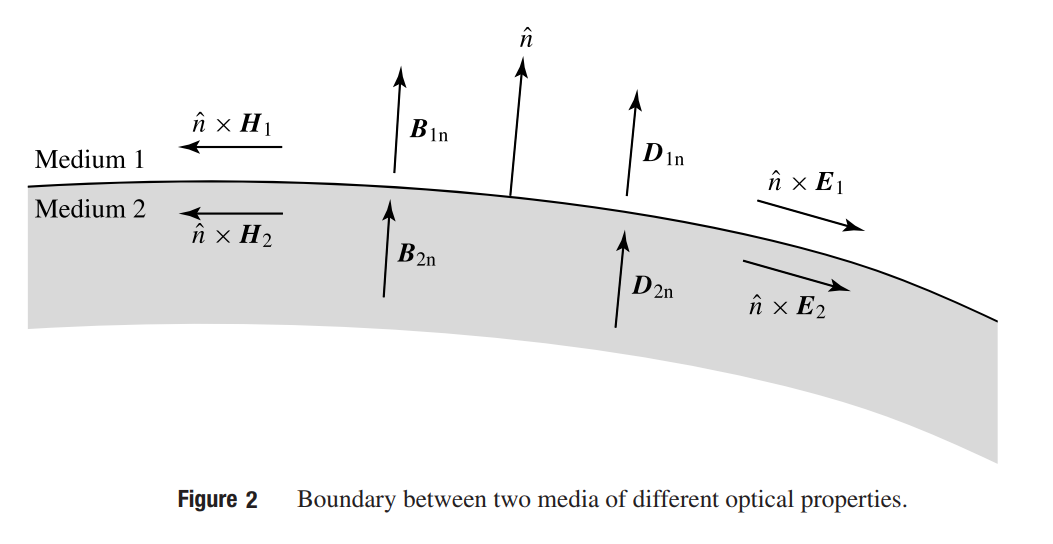These boundary conditions can be derived from Maxwell's equations given in (10) - (13) above. From equation (10) and (11), the tangential components of the fields at the boundary satisfywhere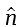is the unit vector normal to the interface as shown in figure 2 above.

From equations (12) and (13), we havefor the normal components of the fields.

The tangential components of E and H must be continuous across an interface, while the normal components of D and B are continuous. Because Bμ0H for optical fields, as discussed above, (18) and (20) also implies that the tangential component of B and the normal component of H are also continuous. Consequently, all of the magnetic field components in an optical field are continuous across a boundary. Possible discontinuities in an optical field exist only in the normal component of E or the tangential component of D

Optical Power and Energy

By multiplying E by (6) and multiplying H by (5), we obtain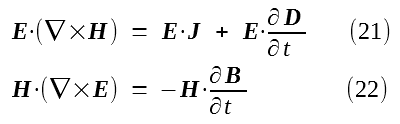Using the vector identitywe can combine (21) and (22) to haveBy using (1) and (2) and rearranging (24), we obtainRecall that power in an electric circuit is given by voltage times current and has the unit of W = V A (watts = volts x amperes). In an electromagnetic field, we find similarly that E · J is the power density that has the unit of V A m-3 or W m-3. Therefore, the total power dissipated by an electromagnetic field in a volume V is just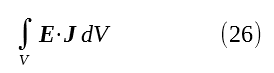Expressing (25) in an integral form, we have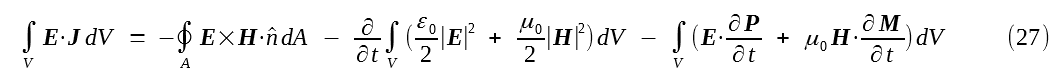where the first term on the right-hand side is a surface integral over the closed surface A of volume V andis the outward-pointing unit normal vector of the surface, as shown in figure 3 below.Clearly, each term in (27) has the unit of power. Each has an important physical meaning. The vector quantity

S = E × H                                            (28)

is called the Poynting vector of the electromagnetic field. It represents the instantaneous magnitude and direction of the power flow of the field.

The scalar quantityhas the unit of energy per unit volume and is the energy density stored in the propagating field. It consists of two components, thus accounting for energies stored in both electric and magnetic fields at any instant of time.

The last term in (27) also has two components associated with electric and magnetic fields, respectively. The quantityis the power density expended by the electromagnetic field on the polarization. It is the rate of energy transfer from the electromagnetic field to the medium by inducing electric polarization in the medium.

Similarly, the quantityis the power density expended by the electromagnetic field on the magnetization.

With these physical meanings attached to these terms, it can be seen that (27) simply states the law of conservation of energy in any arbitrary volume element V in the medium. The total energy in the medium equals that in the propagating field plus that in the electric and magnetic polarization.

In the special case of a linear, nondispersive medium where ε(r - r', t - t') = εδ(r - r')δ(t - t'), (16) simply reduces to D(r, t) = ε · E(r, t). Then, instead of (25), we havefrom (24). In this situation, the total energy density stored in the medium, including that in the propagating field and that in the polarizations, is simply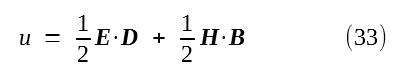For an optical field, J = 0 and M = 0, as is discussed above. Then, (27) becomes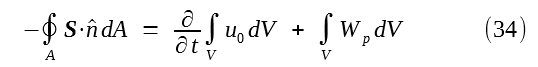which states that the total optical power flowing into volume V through its boundary surface A is equal to the rate of increase with time of the energy stored in the propagating fields in V plus the power transferred to the polarization of the medium in this volume.

In a linear, non-dispersive medium, we haveWave Equation

By applying ∇× to (10) and using (14) and (11), we haveUsing (1), (36) can be expressed aswhereis the speed of light in free space. The wave equation in (37) describes the space-and-time evolution of the electric field of the optical wave. Its right-hand side can be regarded as the driving source for the optical wave. The polarization in a medium drives the evolution of an optical field. This wave equation can take on various forms depending on the characteristics of the medium, as will be seen on various occasions later. For now, we leave it in this general form.

The next part continues in the harmonic fields tutorial.